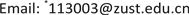1. 前言

2. 物理模型

3. 模拟方法3.1. 方法概述

Yu等人近年来提出了体积格子玻尔兹曼方法(VLBM)，并通过验证证实VLBM方法对于解决如多孔介质、移动边界等复杂的结构模型具有一定的优势。通过引入一个定义为固体基质部分占单元格体积比例的体积参数 P ( x , t ) 来描述多孔介质中基质与孔隙部分，并将多孔介质中单元格分成三类：1) 固体单元格 ( P ( x , t ) = 1 ) ；2) 流体单元格 ( P ( x , t ) = 0 ) ；3) 边界单元格点 ( 0 < P ( x , t ) < 1 ) ，如图4所示。

3.2. 方法介绍

n i ( x , t ) = f i ( x , t ) × [ 1 − P ( x , t ) ] × V (1)

n i ( x + e i Δ t , t + Δ t ) = n i ( x , t ) + Ω i ( x , t ) (2)

Ω i ( x , t ) = − 1 / τ [ n i ( x , t ) − n i e q ( x , t ) ] (3)

n i e q ( x , t ) = N ( x , t ) ω i [ 1 + e i ⋅ u c s 2 + ( e i ⋅ u ) 2 2 c s 4 + ( u ) 2 2 c s 2 ] (4)

n ′ i ( x , t ) = n i ( x , t ) + Ω i ( x , t ) (5)

n ″ i ( x , t + Δ t ) = [ 1 − P ( x , t ) ] n ′ i ( x − e i Δ t , t ) + P ( x − e i * Δ t , t ) n ′ i * ( x i , t ) (6)

ρ ( x , t ) = ​ ∑ n i ( x , t ) / [ 1 − P ( x , t ) ] (7)

u ( x , t ) = ∑ e i n i ( x , t ) / ∑ n i ( x , t ) (8)

p ( x , t ) − p 0 = c s 2 [ ρ ( x , t ) ] − ρ 0 (9)

4. 结果讨论和分析

4.1. 过滤体孔隙度对流场的影响

4.2. 过滤体厚度对流场的影响

30层的过程中，进出口孔道压差从200多pa一直上升到3000多pa。当孔隙度为0.8时，随着过滤体厚度的增加，压差也随之上升。由于孔隙度较大，与孔隙度为0.4的情况相比，进出口孔道之间的压差要小很多，即使当厚度达到30层，压差也仅仅只有60多pa。综上所述，多孔介质过滤体厚度大小对微粒捕集器流线形状影响较小，但是随着过滤体厚度的增加，进出口孔道压差随之上升，因此在微粒捕集器结构的设计优化中，也应该考虑到过滤体厚度。

4.3. 进口速度对于流场的影响

5. 总结与展望